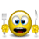TradingView5592 views
Multiple moving averages with same interval in candle bar smoothness over multiple time frames.
option to show/hide the level of resolution for the mtf's default shows 1 ma can go up to 8th resolution.
option for manual input timeframes and configure ma.
Open-source script

In true TradingView spirit, the author of this script has published it open-source, so traders can understand and verify it. Cheers to the author! You may use it for free, but reuse of this code in a publication is governed by House Rules. You can favorite it to use it on a chart.

Want to use this script on a chart?
```study("[RS]MTF Multiple Moving Averages V0", overlay=true)
showTF = input(1, minval=1, maxval=8)
tf1 = input('15')
tf2 = input('30')
tf3 = input('60')
tf4 = input('240')
tf5 = input('D')
tf6 = input('W')
tf7 = input('M')
tf8 = input('12M')

ma_src = input(close)
ma_length = input(4)

mtfma1 = showTF < 1 ? na : security(tickerid, tf1, sma(ma_src, ma_length))
mtfma2 = showTF < 2 ? na : security(tickerid, tf2, sma(ma_src, ma_length))
mtfma3 = showTF < 3 ? na : security(tickerid, tf3, sma(ma_src, ma_length))
mtfma4 = showTF < 4 ? na : security(tickerid, tf4, sma(ma_src, ma_length))
mtfma5 = showTF < 5 ? na : security(tickerid, tf5, sma(ma_src, ma_length))
mtfma6 = showTF < 6 ? na : security(tickerid, tf6, sma(ma_src, ma_length))
mtfma7 = showTF < 7 ? na : security(tickerid, tf7, sma(ma_src, ma_length))
mtfma8 = showTF < 8 ? na : security(tickerid, tf8, sma(ma_src, ma_length))

plot(mtfma1, style=cross, color=close >= mtfma1 ? green : maroon, linewidth=2, join=true)
plot(mtfma2, style=cross, color=close >= mtfma2 ? green : maroon, linewidth=2, join=true)
plot(mtfma3, style=cross, color=close >= mtfma3 ? green : maroon, linewidth=2, join=true)
plot(mtfma4, style=cross, color=close >= mtfma4 ? green : maroon, linewidth=2, join=true)
plot(mtfma5, style=cross, color=close >= mtfma5 ? green : maroon, linewidth=2, join=true)
plot(mtfma6, style=cross, color=close >= mtfma6 ? green : maroon, linewidth=2, join=true)
plot(mtfma7, style=cross, color=close >= mtfma7 ? green : maroon, linewidth=2, join=true)
plot(mtfma8, style=cross, color=close >= mtfma8 ? green : maroon, linewidth=2, join=true)
```

## CommentsHi, Ricardo.

This indicator looks great.

I was wondering if you can help me out with MTF MAs, just regular ones, but with ability to have exponential or standard.
I need three to four MAs in one...just like a regular indicator but for MTF, just like what you did for this indicator.

I hope I make sense.
ReplyHi Ricardo, I like the idea! Awesome work man!

What brought me to this was a search for multiple moving averages in multiple time frames.

Could you code this separately for me: Similar idea to what you are using but instead of showing just one MA (i.e. length=10) and other many other time frames for jsut that one MA, I'd be interested in seeing 5 separate MAs and the time frame of my choosing.

For example, on my chart, I'd like to see the 10, 21, 55, 100, 200 MA in both Daily and Hourly timeframe. No need to get fancy with the colour coding as I'd make the Daily and Hourly the same colour, but the Daily would have a thicker line (I guess you can make all these things user adjustable).

So quite similar to what you have already done, but instead of looking at only one MA in multiple resolutions, we would be able to look at many MAs in however many time frames we selected for that MA (e.g. we could choose to see 100 period MA in 10M, 240M and W; 21 period MA in 15M, 30M 60M; 200 period MA in D, W, M).

Does this make sense?
ReplyCharles
@Charles, try this as a framework and you can expand it as you like:

study('MTF MA' )
src = input(close)
length = input(10)
tf = input('D' )
ma = sma(src, length)
tf_ma = security(tickerid, tf, ma)
plot(tf_ma)
Reply